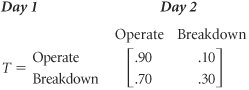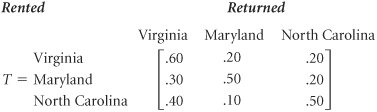Additional Examples of Markov Analysis

[Page F-12]

Although analyzing brand switching is probably the most popular example of Markov analysis, this technique does have other applications. One prominent application relates to the breakdown of a machine or system (such as a computer system, a production operation, or an electrical system). For example, a particular production machine could be assigned the states "operating" and "breakdown." The transition probabilities could then reflect the probability of a machine's either breaking down or operating in the next time period (i.e., month, day, or year).

A machine breakdown example.

As an example, consider a machine that has the following daily transition matrix:The steady-state probabilities for this example are

.88 = steady-state probability of the machine's operating

.12 = steady-state probability of the machine's breaking down

Now if management decides that the long-run probability of .12 for a breakdown is excessive, it might consider increasing preventive maintenance, which would change the transition matrix for this example. The decision to increase maintenance would be based on the cost of the increase versus the value of the increased production output gained from having fewer breakdowns.

Thus far in our discussion of Markov analysis, we have considered only examples that consisted of two states. This has been partially a matter of convenience because 2 x 2 matrices are easier to work with than matrices of a higher magnitude. However, examples that contain a larger number of states are analyzed in the same way as our previous examples. For example, consider the Carry-All Rental Truck Firm, which serves three states Virginia, North Carolina, and Maryland. Trucks are rented on a daily basis and can be rented and returned in any of the three states. The transition matrix for this example follows :A rental truck firm with three states.

The steady-state probabilities for this example are determined by using the same algebraic approach presented earlier, although the mathematical steps are more lengthy and complex. Instead of solving three simultaneous equations, we solve four.

The steady-state probabilities for this example are

 Virginia Maryland North Carolina [.471 .244 .285]

[Page F-13]

Thus, in the long run, these percentages of Carry-All trucks will end up in the three states. If the company had 200 trucks, then it could expect to have the following number of trucks available in each state at any time in the future:

 Virginia Maryland North Carolina [94 49 57]Introduction to Management Science (10th Edition)
ISBN: 0136064361
EAN: 2147483647
Year: 2006
Pages: 358

Similar book on Amazon